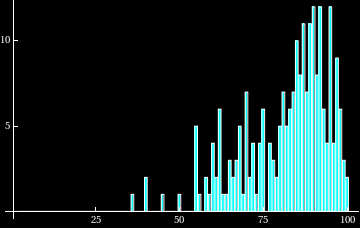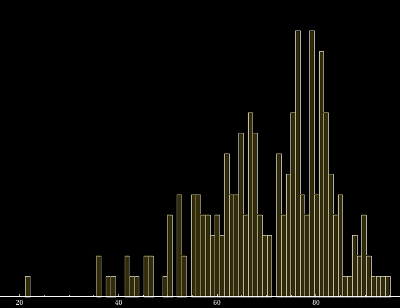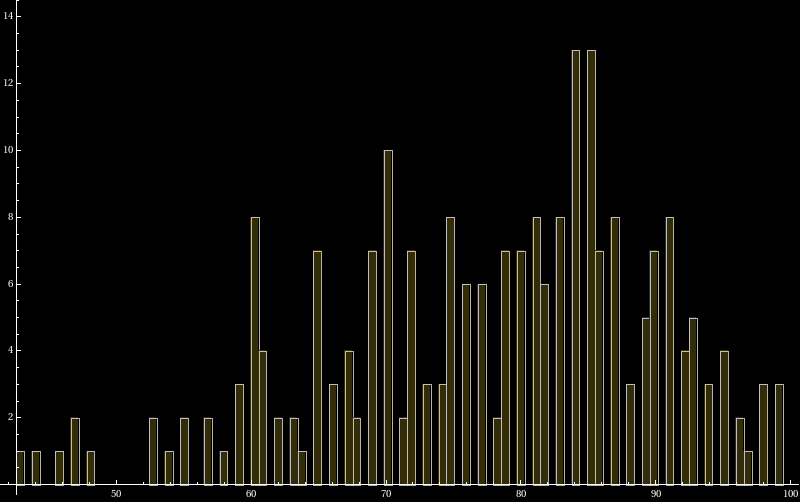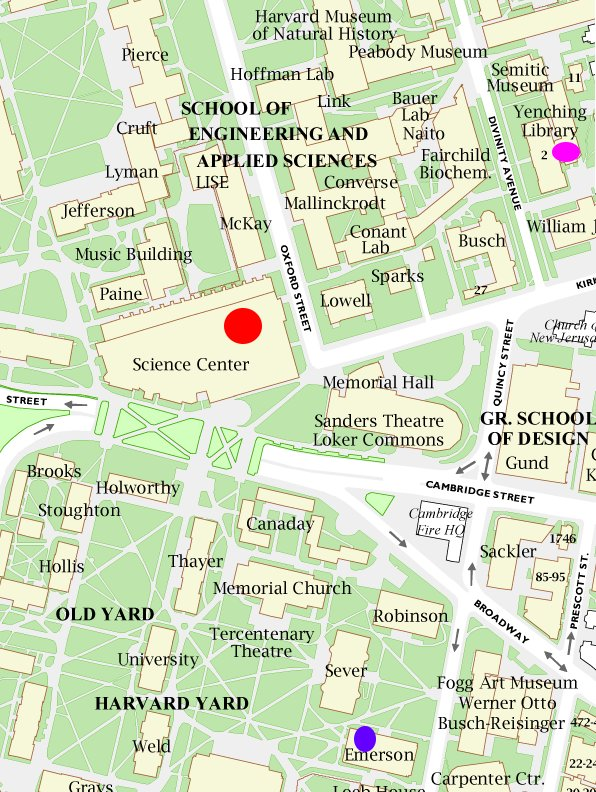M A T H 2 1 B
Mathematics Math21b Spring 2010
Linear Algebra and Differential Equations
Exams
Office: SciCtr 434Our final 21B Exam (B=2, 21+2=23) took place on Saturday, 5/8/10 (5+8+10=23) at 9 AM (A=1,M=13, 9+1+13=23) in Science Center B. There was a final review on Tuesday, 5/4 from 3:30 to 5:03 PM (5+4+3+3+5+3=23) in SC A (S=19,C=3,A=1, 19+3+1=23). The final exam with Solutions. The average of the exam was 80 with a standard deviation of 12. (80/12 = 20/3, 20+3 = 23). The 23 occurrence is no accident as the movie The Number 23 or the movie 23 show.

 Practice exam I [PDF] Solution practice exam 1 Practice exam II [PDF] Solution practice exam 2 Practice exam III [PDF] Solution practice exam 3 Practice exam IV [PDF] Solution practice exam 4 Practice exam V [PDF] Solution practice exam 5 Practice exam VI [PDF] Solution practice exam 6
 Errata (corrected at the indicated time in the above texts): April 29, 1 PM, 2010: Exam 1, Problem 5: [2,1,4] instead of [1,2,4], Problem 8, w2=[1,1,2]/sqrt(6) Problem 10a term missing. Thanks to Mishal Rahman for the corrections. May 1, 8 AM, 2010: Exam 1: Problem 10 c). "t"s missing in the solution. Thanks to Willie Yao for the correction. May 1, 2 PM, 2010: Exam 1: Problem 5). IN the formula for the least square solution, an additional A has slipped in. The actual solution does not use it. Thanks to Han Yan for pointing this out. May 2, 2 PM, 2010, Exam 3, Problem 8), Second column last entry of S is zero. Thanks to Riju Agrawal for the correction May 2, 6 PM, 2010, Exam 1, TF Problem 4. f(x)=x should be changed to f(x)=x2 in order to have a counter example. Thanks to Matthias Wickenburg. May 3, 7 AM, 2010, Exam 1, TF Problem 13 bad explanation. Could use T(2 I)) = 2 T(I) is not true. Thanks to Phillip Yao May 3, 10 PM, 2010, Exam 5, Problem 13, signs under the square root should all be positive. Thanks to Riju Agrawal. May 5, 7 AM, 2010, Exam 4, Problem 8, eigenvalues and eigenvectors mismatch. Final result is ok. Thanks to Sarvagna Patel May 5, 7 AM, 2010, Exam 6, Problem 14, a cosh should have been cos. Thanks to Lee Seligman and Pim Valantagul. May 5, 11 AM, 2010, Exam 3, Problem 3e) a minus sign in the coefficient of w1. Thanks to Christine Shrock. May 5, 11:50 PM, 2010, Exam 4, Problem TF18. The matrix should have a -1 in the upper right corner. Thanks to Arjun Nandkishore. May 6, 6 PM, 2010, Exam 1, Problem 13, one Sin(ny) should have been sin(my). Thanks to Talya Fox May 6, 9 PM, 2010, Exam 1, Problem 10c) a t missing in a cos term. Thanks to Rekha Auguste-Nelson May 6, 9 PM, 2010, Exam 1, Problem 12a) signs missing in simplification of Fourier exponents. Thanks to Riju Agrawal. May 7, 8 AM, 2010, Exam 2, TF 17, we have to assume that the diagonal entries are positive. Thanks to Lee Seligman. May 7, 12 AM, 2010, Exam 6, Problem 2c, The eigenvalues are -4,1. Thanks to Riju Agrawal.The 2. hourly took place on Tuesday, April 6th from 7 -8:30 PM. We had a global review session in Hall C from 7-8:30 PM on Sunday April 4th. For the midterm, we use the two lecture halls for the exam as for the first midterm: Yenching Auditorium, 2 Divinity Ave., #18 (Last Names A-K) and Emerson 105 (last names L-Z). The exam appeared tougher than the first midterm. The average was 70. The standard deviation was 13. The exam [PDF], Solution draft [PDF].

 Practice exam I [PDF] Solution practice exam 1 Practice exam II [PDF] Solution practice exam 2 Practice exam III [PDF] Solution practice exam 3 Practice exam IV [PDF] Solution practice exam 4 Practice exam V [PDF] Solution practice exam 5 Practice exam VI [PDF] Solution practice exam 6

 Errata (corrected at the indicated time in the above texts): March 30, 11 PM, 2010: Exam 1, Problem 6: c2=-1. Thanks to Matthew Tung for the correction. April 3, 8 PM, Exam 3, the solution to Problem 5b was missing. Thanks to Chandan Lodha for noticing. April 3, 5 PM, Exam 8, in the Harvard-Yale problem the story does not quite match the matrix. One would have to use the transpose matrix. We do not change the problem for now. Thanks to Matthew Tung. April 3, 3 PM, Exam 5, Problem 5, The final vector should be [-2,0,1]. Thanks to Matthew Tung April 3, 11PM, Exam 1, Problem 8, there had been a spurious 3 in the lower left corner of the R matrix. Thanks to Scott Crouch. April 4, 12 PM, Exam 1, problem 6, c1=1,c2=-1. not c1=c2=-1. The final result was right. Thanks to Kelsey Romatoski. April 5, 10 PM, Exam 2, Problem 2, due to the recent restriction to 3D, the box with det(A)=-1 for reflection at a line should not be applied, Thanks to Sam Himel. April 5, 11 PM, Exam 6, Problem 6, typo in lowest right entry of R matrix. Thanks to Scott Crouch and Kelsey Romatoski.The first hourly took place on Tuesday, March 2nd from 7 -8:30 PM. The exam took place in two lecture halls: Yenching Auditorium, 2 Divinity Ave., #18 (Last Names A-K) and Emerson 105 (last names L-Z) on both March 2nd, 7-8:30. We had a global review on Sunday, February 28, 2010 from 7-8:30 PM in Hall C. The average of the exam was 76. The standard deviation 12. The exam [PDF], Solutions [PDF]

 Practice exam I [PDF] Solution practice exam 1 Practice exam II [PDF] Solution practice exam 2 Practice exam III [PDF] Solution practice exam 3 Practice exam IV [PDF] Solution practice exam 4 Practice exam V [PDF] Solution practice exam 5Errata (corrected at the indicated time in the above texts): February 27, 11 AM, 2010: Exam 1, Problem 10: confusion between Anna (vector a) and Bob (vector b). Thanks to Phil Yao. February 27, 1 PM, 2010: Exam 3, TF Problem 15: Explanation had a "True" which did not fit with the checked "False", Problem 7a) solution typo in kernel element. Thanks to Alvin Siu February 28, 3 PM, 2010: Exam 1, Problem 7. x = r - s (not x = -r -s), the end solution is correct. Thanks to Carl Daher. March 1, 7 PM, 2010, Exam 1, Problem 4). In an intermediate row reduction step, a 1 should have been zero. End result is unaffected. Thanks to Sam Himmel March 1, 10 PM, 2010, Exam 2, Problem 7) The row reduced matrix has a 0 in the upper right corner. Thanks to Ainsley Faux March 1, 11 PM, 2010, Exam 3, Problem 3c, a solution vector had changed from [3 1 0 0] to the wrong [3 0 1 0]. Thanks to Chandan Lodha. March 4, 6 PM, Exam 6, Problem 6b. Last element in S was incorrect in QR factorization. Thanks to Matthew Tung. March 5, 9 AM, Exam 5, TF 5, should be 2P - I, Thanks to Christine Shrock.

 Official policy at Harvard is that "out of sequence midterm exams" are only offered for students observing religious holidays. We sometimes can accommodate students who happen to have an important sports event at the same time (it must be an actual game or tournament) or a concert or theater event (regular practice or rehearsals of course do not qualify to miss the exam). In case of a qualifying conflict, contact the course head to see whether it is possible to arrange the exam earlier at the same day. We will need a letter from your coach or director. The request has to come at least a week before the exam.